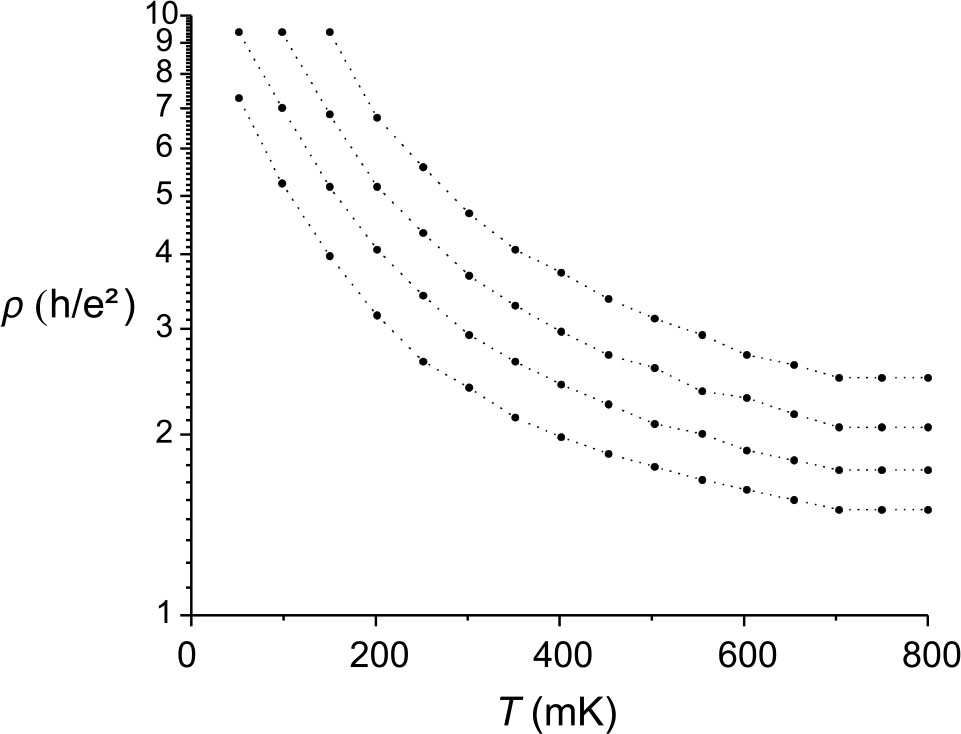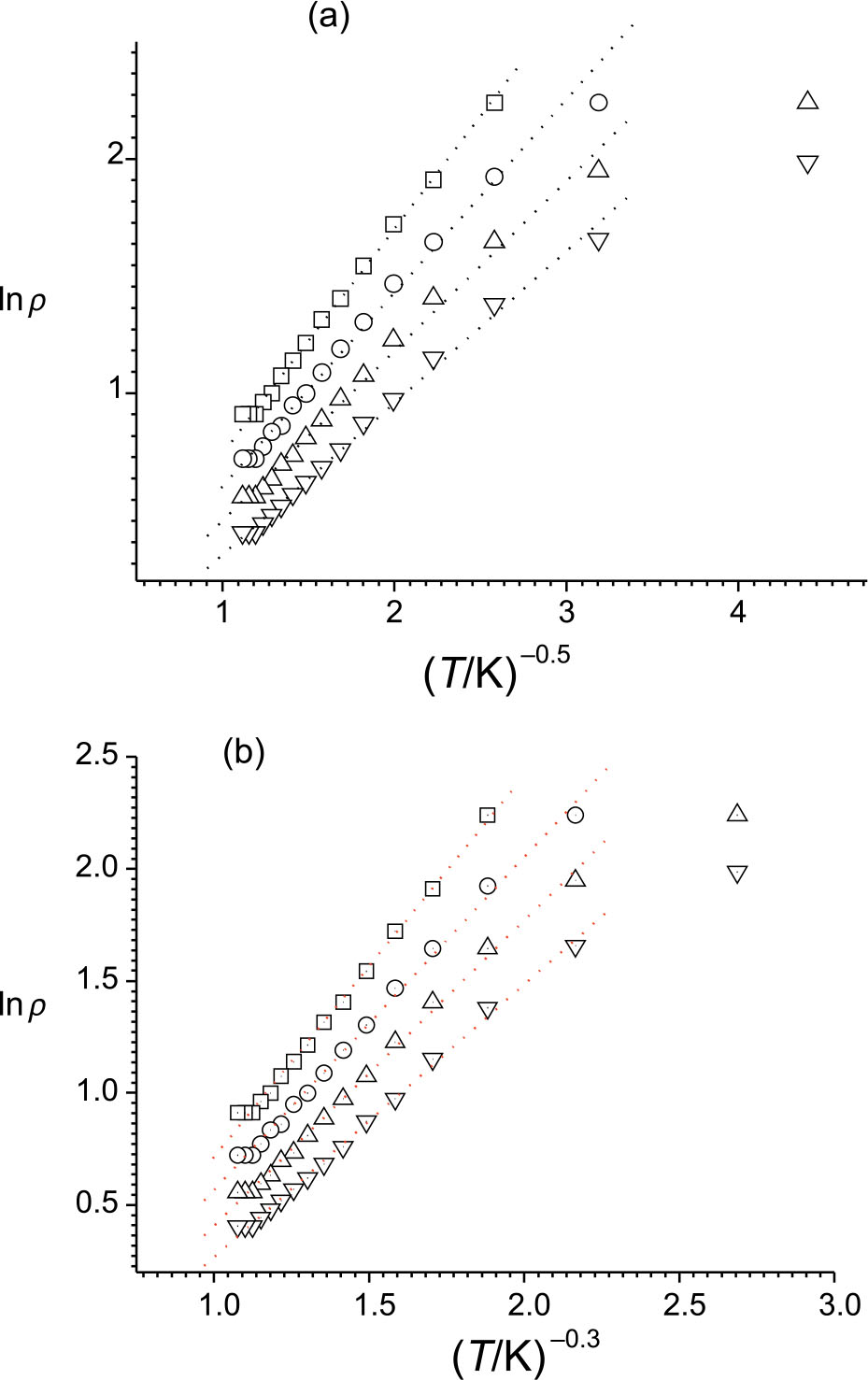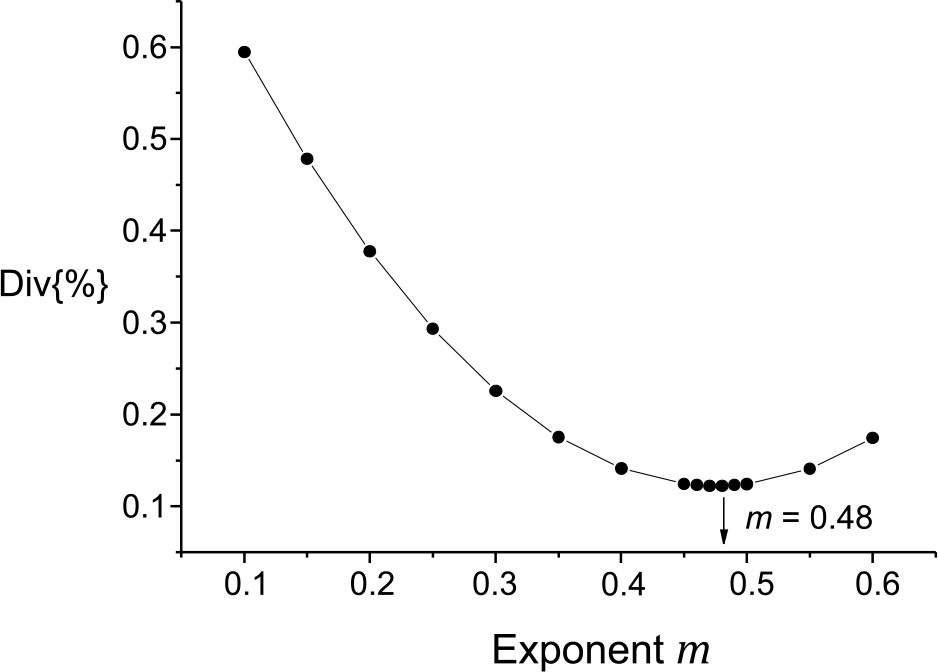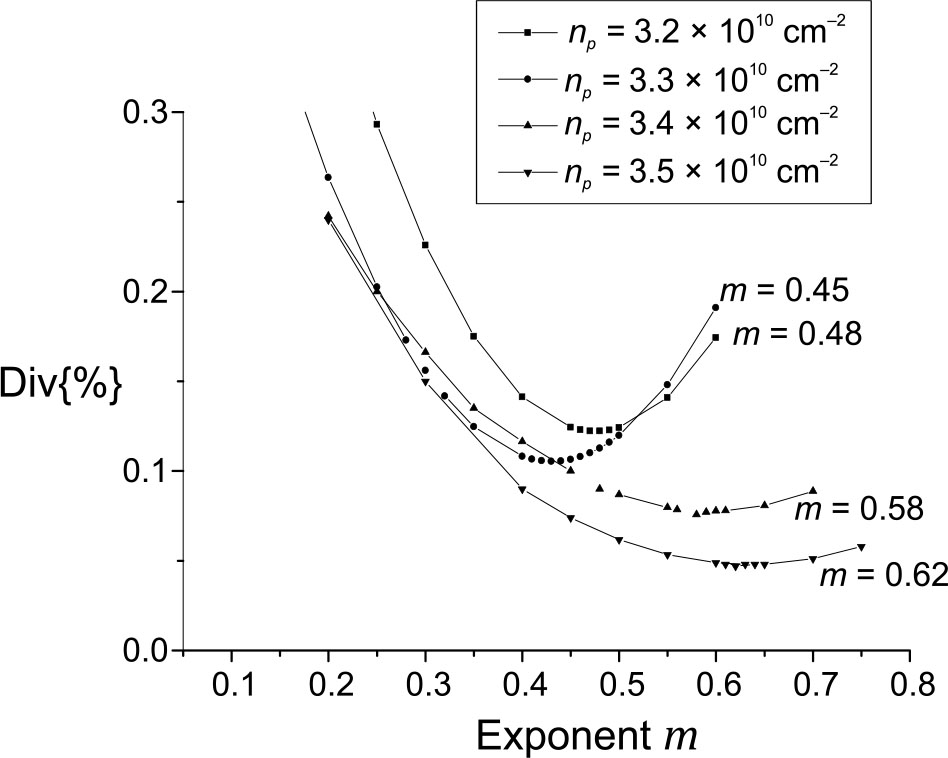# EFROS–SHKLOVSKII HOPPING IN THE ELECTRONIC TRANSPORT IN 2D p-GaAs

S. Dlimia,

L. Limounya

J. Hemineb,

A. Echchelhc,

A. El kaaouachia

a Physics Department, Faculty of Sciences, University Ibn Zohr, B. P 8106, Hay Dakhla, 80000 Agadir, Morocco

b Laboratoire des systèmes électroniques, traitement de l’information, mécanique et énergétique, Faculté des Sciences, Université Ibn Tofaïl, Kénitra, Morocco

c Laboratory of Energetic Engineering and Materials, Faculty of Sciences, Ibn Tofaïl University, Kénitra, Morocco
Email: dlimi1975@gmail.com

Received 16 May 2020; revised 4 June 2020; accepted 17 June 2020

We have investigated the resistivity of a 2D hole system in GaAs in the temperature range 200 mK < T < 800 mK at zero magnetic field and low hole densities when the system is near the metal–insulator transition in the insulating side. We have found that the resistivity follows the Efros–Shklovskii variable range hopping (ES-VRH) law, this behaviour is consistent with the existence of a Coulomb gap. The resistivity scales with temperature and the prefactor has been found independent of temperature and density, thus confirming the dominance of hole–hole interaction.

Keywords: Coulomb gap, variable range hopping, 2D GaAs hole system, low temperature

PACS: 73.21.Fg, 73.40.−c, 73.90.+f

### 1. Introduction

For several decades, condensed matter physicists have been able to build two-dimensional quantum systems, such as Si-MOSFETs, or GaAs/GaAlAs semiconductor heterostructures. In these systems, the idea is to trap electrons in a very thin potential well at the interface between two semiconductors (or between a semiconductor and an insulator), so that the movement of the carriers becomes strictly two-dimensional, in the plane of the interface. As the manufacturing processes are not yet perfect, impurities and defects are randomly distributed in the material, hence the presence of a disorder potential in which the electrons move, correlated with each other by the Coulomb interaction.

In a highly disordered electronic system, each electron (trapped by the disorder) is assumed to be located at a single site, so that overlap between wave functions can be neglected. This brings us back to a classic problem, without kinetic energy. In the absence of interaction, the system has a constant density of states. On the contrary, in the presence of interactions, the state density ρ(E) close to the Fermi level is decreased according to Eq. (1):

$\rho \left(E\right)\sim {\left(E-{E}_{F}\right)}^{d-1}\text{\hspace{0.17em}}\text{\hspace{0.17em}}\text{\hspace{0.17em}}\text{\hspace{0.17em}}\text{\hspace{0.17em}}\text{\hspace{0.17em}}\text{\hspace{0.17em}}\text{\hspace{0.17em}}\text{\hspace{0.17em}}\text{\hspace{0.17em}}\text{\hspace{0.17em}}\text{\hspace{0.17em}}\text{\hspace{0.17em}}\text{\hspace{0.17em}}\text{\hspace{0.17em}}\text{\hspace{0.17em}}\text{\hspace{0.17em}}\text{\hspace{0.17em}}\text{\hspace{0.17em}}\text{\hspace{0.17em}}\text{\hspace{0.17em}}\text{\hspace{0.17em}}\text{\hspace{0.17em}}\text{\hspace{0.17em}}\text{\hspace{0.17em}}\text{\hspace{0.17em}}\text{\hspace{0.17em}}\text{\hspace{0.17em}}\text{\hspace{0.17em}}\text{\hspace{0.17em}}\text{\hspace{0.17em}}\text{\hspace{0.17em}}\text{\hspace{0.17em}}\text{\hspace{0.17em}}\text{\hspace{0.17em}}\text{\hspace{0.17em}}\text{\hspace{0.17em}}\text{\hspace{0.17em}}\text{\hspace{0.17em}}\text{\hspace{0.17em}}\text{\hspace{0.17em}}\text{\hspace{0.17em}}\text{\hspace{0.17em}}\text{\hspace{0.17em}}\text{\hspace{0.17em}}\text{\hspace{0.17em}}\text{\hspace{0.17em}}\text{\hspace{0.17em}}\text{\hspace{0.17em}}\text{\hspace{0.17em}}\text{\hspace{0.17em}}\text{\hspace{0.17em}}\left(1\right)$

Here d is the dimension of space. In other words, interactions deplete the Fermi level. This is called the Coulomb gap, even if it is not a real gap since the density of states cancels only at the Fermi level (E = EF).

The Coulomb gap is detrimental to the transport properties of the system. More precisely, Mott’s law  giving the ρ(T) dependence of resistivity as a function of temperature in Anderson’s insulator, without interaction, must be corrected. Whatever the dimension d, this law becomes

$\rho \left(T\right)\sim \text{exp}{\left(\frac{{T}_{0}}{T}\right)}^{1/2},\text{\hspace{0.17em}}\text{\hspace{0.17em}}\text{\hspace{0.17em}}\text{\hspace{0.17em}}\text{\hspace{0.17em}}\text{\hspace{0.17em}}\text{\hspace{0.17em}}\text{\hspace{0.17em}}\text{\hspace{0.17em}}\text{\hspace{0.17em}}\text{\hspace{0.17em}}\text{\hspace{0.17em}}\text{\hspace{0.17em}}\text{\hspace{0.17em}}\text{\hspace{0.17em}}\text{\hspace{0.17em}}\text{\hspace{0.17em}}\text{\hspace{0.17em}}\text{\hspace{0.17em}}\text{\hspace{0.17em}}\text{\hspace{0.17em}}\text{\hspace{0.17em}}\text{\hspace{0.17em}}\text{\hspace{0.17em}}\text{\hspace{0.17em}}\text{\hspace{0.17em}}\text{\hspace{0.17em}}\text{\hspace{0.17em}}\text{\hspace{0.17em}}\text{\hspace{0.17em}}\text{\hspace{0.17em}}\text{\hspace{0.17em}}\text{\hspace{0.17em}}\text{\hspace{0.17em}}\text{\hspace{0.17em}}\text{\hspace{0.17em}}\text{\hspace{0.17em}}\text{\hspace{0.17em}}\text{\hspace{0.17em}}\text{\hspace{0.17em}}\text{\hspace{0.17em}}\text{\hspace{0.17em}}\text{\hspace{0.17em}}\text{\hspace{0.17em}}\text{\hspace{0.17em}}\text{\hspace{0.17em}}\left(2\right)$

where T0 is the characteristic temperature. Eq. (2) is known as Efros–Shklovskii’s law.

Finally, taking kinetic energy into account can lead to the closure of the Coulomb gap if quantum fluctuations compensate for the effects of interactions. Typically, closure of the Coulomb gap is expected for a value of the interaction parameter rs ~ 1 . Thus in Anderson’s insulator, the temperature dependence of resistivity is given by Mott’s law described by

$\rho \left(T\right)\sim \text{exp}{\left(\frac{{T}_{0}}{T}\right)}^{1/\left(d-1\right)},\text{\hspace{0.17em}}\text{\hspace{0.17em}}\text{\hspace{0.17em}}\text{\hspace{0.17em}}\text{\hspace{0.17em}}\text{\hspace{0.17em}}\text{\hspace{0.17em}}\text{\hspace{0.17em}}\text{\hspace{0.17em}}\text{\hspace{0.17em}}\text{\hspace{0.17em}}\text{\hspace{0.17em}}\text{\hspace{0.17em}}\text{\hspace{0.17em}}\text{\hspace{0.17em}}\text{\hspace{0.17em}}\text{\hspace{0.17em}}\text{\hspace{0.17em}}\text{\hspace{0.17em}}\text{\hspace{0.17em}}\text{\hspace{0.17em}}\text{\hspace{0.17em}}\text{\hspace{0.17em}}\text{\hspace{0.17em}}\text{\hspace{0.17em}}\text{\hspace{0.17em}}\text{\hspace{0.17em}}\text{\hspace{0.17em}}\text{\hspace{0.17em}}\text{\hspace{0.17em}}\text{\hspace{0.17em}}\text{\hspace{0.17em}}\text{\hspace{0.17em}}\text{\hspace{0.17em}}\text{\hspace{0.17em}}\text{\hspace{0.17em}}\text{\hspace{0.17em}}\text{\hspace{0.17em}}\text{\hspace{0.17em}}\text{\hspace{0.17em}}\text{\hspace{0.17em}}\text{\hspace{0.17em}}\text{\hspace{0.17em}}\text{\hspace{0.17em}}\text{\hspace{0.17em}}\text{\hspace{0.17em}}\left(3\right)$

where T0 is the characteristic temperature.

The typical distance of hopping can be evaluated by

${r}_{M}=a{\left(\frac{{T}_{0}}{T}\right)}^{\frac{1}{d+1}},\text{\hspace{0.17em}}\text{\hspace{0.17em}}\text{\hspace{0.17em}}\text{\hspace{0.17em}}\text{\hspace{0.17em}}\text{\hspace{0.17em}}\text{\hspace{0.17em}}\text{\hspace{0.17em}}\text{\hspace{0.17em}}\text{\hspace{0.17em}}\text{\hspace{0.17em}}\text{\hspace{0.17em}}\text{\hspace{0.17em}}\text{\hspace{0.17em}}\text{\hspace{0.17em}}\text{\hspace{0.17em}}\text{\hspace{0.17em}}\text{\hspace{0.17em}}\text{\hspace{0.17em}}\text{\hspace{0.17em}}\text{\hspace{0.17em}}\text{\hspace{0.17em}}\text{\hspace{0.17em}}\text{\hspace{0.17em}}\text{\hspace{0.17em}}\text{\hspace{0.17em}}\text{\hspace{0.17em}}\text{\hspace{0.17em}}\text{\hspace{0.17em}}\text{\hspace{0.17em}}\text{\hspace{0.17em}}\text{\hspace{0.17em}}\text{\hspace{0.17em}}\text{\hspace{0.17em}}\text{\hspace{0.17em}}\text{\hspace{0.17em}}\text{\hspace{0.17em}}\text{\hspace{0.17em}}\text{\hspace{0.17em}}\text{\hspace{0.17em}}\text{\hspace{0.17em}}\text{\hspace{0.17em}}\text{\hspace{0.17em}}\text{\hspace{0.17em}}\text{\hspace{0.17em}}\text{\hspace{0.17em}}\text{\hspace{0.17em}}\text{\hspace{0.17em}}\text{\hspace{0.17em}}\text{\hspace{0.17em}}\text{\hspace{0.17em}}\text{\hspace{0.17em}}\text{\hspace{0.17em}}\text{\hspace{0.17em}}\text{\hspace{0.17em}}\left(4\right)$

where a is the localization length and ${T}_{0}=\frac{{E}_{0}}{{k}_{B}}$ , where E0 is the average energy difference between neighbouring levels, and kB is Boltzmann’s constant. This typical distance varies as ${T}^{-\frac{1}{d+1}}$ , therefore increases as the temperature decreases, just justifying the denomination of variable range hopping (VRH) for this conduction mechanism.

In this paper, we present the behaviour of hopping resistivity in a strongly localized state near the metal–insulator transition (MIT) and we test the VRH conduction models to determine the dominant hopping mechanism in 2D p-GaAs. The experimental data which we reanalysed was obtained in 2D GaAs hole gas by Hamilton and co-workers . The sample has a peak mobility of µ = 2.5 × 105 cm2 V–1 s–1.

### 2. Results and discussion

Fig. 1 shows the evolution of resistivity as a function of temperature in the insulating state near the insulator–metal transition for densities 3.2 × 1010, 3.3 × 1010, 3.4 × 1010 and 3.5 × 1010 cm–2. We remark that the resistivity increases monotonically when the temperature decreases $\left(\frac{\text{d}\rho }{\text{d}T}<0\right)$ , and seems to tend towards infinity at zero temperature, which is characteristic of an insulator [4, 6].Fig. 1. The resistivity versus temperature for the GaAs heterostructure at different carrier densities.

At low density and for independent electrons, we are in the limit kFle < 1 and a semi-classical model cannot be applied. The theory of transport between localized states by variable range hopping gives precise predictions on the expected temperature dependence. To test the validity of these models in our case, the ρ(T) curves have been fitted by the law 

where ρ0 is the prefactor, T0 is the characteristic temperature and m is the characteristic exponent the value of which distinguishes different hopping mechanism.

The important parameter of this law is the exponent m, which makes it possible to determine the transport mechanism of carriers in the insulating phase. The curves in Fig. 2 show the resistivity as a function of Tm without distinguishing between the both hopping mechanisms (ES-VRH and Mott-VRH). In this representation, the hopping law with the exponent m and the temperature-independent prefactor ρ0 gives a straight line for both cases (m = 1/2 and m = 1/3) without possibility to distinguish the best exponent because of a small range of the resistivity explored. These remarks are in agreement with measurements carried out on Si MOSFETs [4, 1113], in p-SiGe  and p-GaAs  heterostructures which have shown that, at low carrier density, the law described in Eq. (5) is well followed with the exponent m = 1/2 (for ≤ T0/4), ). The exponent is therefore the one expected for variable range hopping (VRH) in the presence of a gap due to electron interactions. However, this behaviour is not specific to systems exhibiting the metal–insulator transition since it has been observed in heterostructures that do not exhibit metallic behaviour .Fig. 2. Resistivity versus temperature for both cases: (a) ES-VRH and (b) Mott-VRH.

The values of the prefactor deduced from the fitting of resistivity curves are shown in Fig. 3, and these values are found almost constant. This behaviour is similar to what has been observed in Si MOSFETs [4, 1112], where a universal prefactor independent of temperature and density has been obtained, which is in agreement with the variable range hopping theory. The prefactor is generally temperature dependent. We found in a previous work a value of the prefactor close to the universal value 1 h/e2 .

The prefactor ρ0 obtained in Si MOSFETs [4, 11, 13] is close to h/e2 and independent of temperature and density. In the p-SiGe  and p-GaAs  heterostructures, ρ0.5 h/e2 is independent of density. These observations are inconsistent with a mechanism where the hopping is generated by the electron–phonon interaction, for which the dependence of ρ0 on temperature and density is expected, and can be attributed to another hopping mechanism related to the electron interaction, confirming once again the important role of interactions in the studied systems.

In order to establish the dominant hopping mechanism, we used the method of percentage deviation Dev{%}. This method is widely used in statistics to evaluate the difference between the estimated values (ρi) and the value of linear fits (ρ) [17, 18]

$\text{Dev}\left\{%\right\}=\sqrt{\frac{1}{n}\sum _{i=1}^{i=n}{\left[\frac{100}{{\rho }_{i}}\left(\rho -{\rho }_{i}\right)\right]}^{2}},\text{\hspace{0.17em}}\text{\hspace{0.17em}}\text{\hspace{0.17em}}\text{\hspace{0.17em}}\text{\hspace{0.17em}}\text{\hspace{0.17em}}\text{\hspace{0.17em}}\text{\hspace{0.17em}}\text{\hspace{0.17em}}\text{\hspace{0.17em}}\text{\hspace{0.17em}}\text{\hspace{0.17em}}\text{\hspace{0.17em}}\text{\hspace{0.17em}}\text{\hspace{0.17em}}\text{\hspace{0.17em}}\text{\hspace{0.17em}}\text{\hspace{0.17em}}\text{\hspace{0.17em}}\text{\hspace{0.17em}}\text{\hspace{0.17em}}\text{\hspace{0.17em}}\text{\hspace{0.17em}}\text{\hspace{0.17em}}\left(6\right)$

n being the number of experimental points.

As shown in Figs. 4 and 5, we have obtained values of the exponent 𝑚 close to 1/2 and far from 1/3 which allows us, on the one hand, to exclude the Mott hopping mechanism where the hopping is assisted by phonons [10, 1415] and, on the other hand, to confirm the existence of the Efros–Shklovskii hopping where the hopping is assisted by electrons. The exponent 𝑚 depends on the density, in fact, the more the density approaches the critical density of the insulator–metal transition which is estimated at npc = 4.6 × 1010 cm–2 [6, 19], the more the exponent 𝑚 increases and tends towards the value 𝑚 = 1 that is characteristic of a hopping mechanism of the activated type .Fig. 4. Percentage deviation versus the exponent 𝑚 for the density np = 3.3 × 1010 cm–2.Fig. 5. Percentage deviation versus the exponent 𝑚 for different densities.

### 3. Conclusions

We have shown that it is possible to adjust the resistivity curves ρ(T) with an exponent m = 1/2 or m = 1/3, without being able to differentiate the most exact mechanism. Using the deviation method, we were able to determine the most accurate hopping mechanism.

The results presented in this paper are consistent with the existence of the 2D Coulomb gap where the resistivity follows the Efros–Shklovs-kii law , with a prefactor independent of temperature and density. The resistivity scales with temperature and the prefactor was found to be independent of temperature and density, which confirms the dominance of hole–hole interaction. In brief, the transport in the 2D GaAs hole system is most consistent with the forms for VRH with a Coulomb gap.

### Acknowledgements

We are grateful to Professor A.R. Hamilton who has allowed us to use his experimental results on a dilute 2D GaAs hole system sample.

### References

 A.L. Efros and B.I. Shklovskii, Coulomb gap and low temperature conductivity of disordered systems, J. Phys. C 8, L49 (1975).

 N.F. Mott and E.A. Davis, Electronic Processes in Non-crystalline Materials, 2nd ed. (Clarendon Press, Oxford, 1979).

 A.R. Hamilton, M.Y. Simmons, M. Pepper, E.H. Linfield, and D.A. Ritchie, Localisation and the metal–insulator transition in two dimensions, Physica B 296, 21–31 (2001).

 S.V. Kravchenko, W.E. Mason, G.E. Bowker, J.E. Furneaux, V.M. Pudalov, and M. D’Iorio, Scaling of an anomalous metal-insulator transition in a two-dimensional system in silicon at B=0, Phys. Rev. B 51, 7038–7045 (1996).

 Y. Hanein, U. Meirav, D. Shahar, C.C. Li, D.C. Tsu, and H. Shtrikman, The metallic-like conductivity of a two-dimensional hole system, Phys. Rev. Lett. 80, 1288 (1998).

 S. Dlimi, A. El kaaouachi, R. Abdia, A. Narjis, G. Bis kupski, J. Hemine, L. Limouny, and A. Sybous, Low temperature electrical transport properties in dilute 2D GaAs hole systems with magnetic field, AIP Conf. Proc. 1435, 385 (2012).

 N.F. Mott, Conduction in glasses containing transition metal ions, J. Non-Cryst. Solids 1, 1 (1968).

 T. Ferrus, R. George, C.H.W. Barnes, N. Lumpkin, D.J. Paul, and M. Pepper, Evidence for multiple impurity bands in sodium-doped silicon MOSFETs, Phys. Rev. B 73(4), 041304(R) (2006).

 H. Liu, A. Pourret, and P. Guyot-Sionnest, Mott and Efros-Shklovskii variable range hopping in CdSe quantum dots films, ACS Nano 4, 5211– 5216 (2010).

 S. Dlimi, A. El kaaouachi, A. Narjis, L. Limouny, A. Sybous, and M. Errai, Hopping energy and percolation-type transport in p-GaAs low densities near the 2D metal–insulator transition at zero magnetic field, J. Phys. Chem. Solids 74, 1349–1354 (2013).

 W. Mason, S.V. Kravchenko, G.E. Bowker, and J.E. Furneaux, Experimental evidence for a Coulomb gap in two dimensions, Phys. Rev. 52, 7857 (1995).

 W. Mason, S.V. Kravchenko, and J.E. Furneaux, Experimental evidence of the Coulomb gap in a high-mobility 2D electron system in silicon, Surf. Sci. 361/362, 953 (1996).

 K.M. Mertes, D. Simonian, M.P. Sarachik, S.V. Kravchenko, and M. Klapwijk, Response to parallel magnetic field of a dilute two-dimensional electron system across the metal-insulator transition, Phys. Rev. B 60, R5093 (1999).

 P.T. Coleridge, R.L. Williams, Y. Feng, and P. Zawadzki, Metal-insulator transition at B=0 in p-type SiGe, Phys, Rev. B 56, R12764 (1997).

 M.Y. Simmons, A.R. Hamilton, M. Pepper, E.H. Linfield, P.D. Rose, D.A. Ritchie, A.K. Savchenko, and T.G. Griffiths, Metal-insulator transition at B=0 in a dilute two dimensional GaAs-AlGaAs hole gas, Phys. Rev. Lett. 80, 1292 (1998).

 S.I. Khondaker, I.S. Shlimak, J.T. Nicholls, M. Pepper, and D.A. Ritchie, Two-dimensional hopping conductivity in a δ-doped GaAs/ AlxGa1–xAs heterostructure, Phys. Rev. B 59, 4580 (1999).

 A. Narjis, A. El kaaouachi, L. Limouny, S. Dlimi, A. Sybous, J. Hemine, R. Abdia, and G. Biskupski, Study of insulating electrical conductivity in hydrogenated amorphous silicon–nickel alloys at very low temperature, Physica B 406, 4155–4158 (2011).

 L. Limouny, A. El kaaouachi, R. Abdia, A. Narjis, G. Biskupski, J. Hemine, A. Sybous, and S. Dlimi, Study of electrical resistivity in 2D Si-MOSFETS at very low temperature, AIP Conf. Proc. 1435, 401–408 (2012).

 S. Dlimi, A. El kaaouachi, and A. Narjis, Density inhomogeneity driven metal–insulator transition in 2D p-GaAs, Physica E 54, 181–184 (2013).

 S. Dlimi, A. El kaaouachi, A. Narjis, L. Limouny, A. Sybous, M. Errai, and G. Biskupski, Evidence for the correlated hopping mechanism in p-GaAs near the 2D MIT at B = 0T, J. Optoelectron. Adv. Mater. 15(11–12), 1222–1227 (2013).

## EFROSO IR ŠKLOVSKIO ŠOKAVIMAS ELEKTRONŲ PERNAŠOJE DVIMAČIAME p-GaAs

S. Dlimi a, L. Limouny a, J. Hemine b, A. Echchelh b, A. El Kaaouachi a

a Ibn Zohr universitetas, Agadyras, Marokas

b Ibn Tofaïl universitetas, Kenitra, Marokas